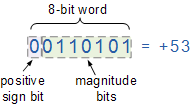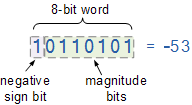# A Clojure Introduction to Binary and Bitwise Operators

I’ve been working in Clojure and ClojureScript lately. A few months back, I came across this one weird trick to master Clojure. TL;DR: it recommends reading the API docs, fully, top to bottom.

I’m not even joking! Go to this URL http://clojure.github.io/clojure/clojure.core-api.html and start reading from top to bottom. If you did not read through that page, you may not know about amap. If you stopped reading before you get to ‘f’ you wouldn’t know about frequencies. However, if you read all the way through, you will be rewarded with knowledge about vary-meta.

In the process of doing this, I was struck by all the `bit-` functions — of the ~600 vars and functions in `clojure.core`, there are 12 specifically for bitwise operations.

### Binary Fundamentals

A quick overview on binary:

Some things to note:

• We can disregard leading zeros in binary, so binary `0010` and binary `10` both represent decimal `2`.
• Each position is one bit (“bit” means “binary digit”). So `1111` is a 4-bit integer (so we can represent up to 15 different values). A 32-bit integer maxes at `11111111111111111111111111111111` (so we can represent up to 4,294,967,295 values). We’ll see that we don’t always use every position counting up from 0 (the left-most position will be used to determine positive/negative).
• This blog post uses `2r....` and `Integer/toBinaryString` to convert between binary and decimal. Other functions and formatters are discussed here.

### Bitwise Operators

This brings us to the bitwise operators. There are 12 in `clojure.core` (all visible in the source):

`bit-and` – Bitwise and

`bit-or` – Bitwise or

`bit-xor` – Bitwise exclusive or

`bit-not` – Bitwise complement

`bit-and-not` – Bitwise and with complement

`bit-clear` – Clear bit at index n

`bit-flip` – Flip bit at index n

`bit-set` – Set bit at index n

`bit-test` – Test bit at index n

`bit-shift-left` – Bitwise shift left

`bit-shift-right` – Bitwise shift right

`unsigned-bit-shift-right` – Bitwise shift right, without sign-extension

### Negative Binary (Signed and Unsigned Integers)

##### Sign-Magnitude NotationSource: “Signed Binary Numbers”

Negative numbers can be represented using signed integers, where the left-most position represents whether the integer is positive or negative. In one implementation (sign-magnitude notation), the left-most bit is the sign, and the remaining bits are the value.

This means that in an `n`-bit integer (e.g., 4-bit), the left-most bit signifies positive/negative, so the remaining `n-1` (3) bits can hold the actual value. There are 16 possible values for a 4-bit integer (`0000` to `1111`), meaning that a signed 4-bit integer can go from -7 to +7 (it is 16 possible values because we can represent both `-0` and `+0`, as binary `1000` and `0000`).

##### Two’s Complement

Two’s Complement is a slightly more complicated scheme for signed integers (though not overly so), and the one more commonly used. A leading `1` still signifies a negative integer. The difference is that it’s not simply sign (1st bit) and magnitude (remaining bits), because the magnitude is determined using the complement (hence the name).

Up until now, all the code samples have been unsigned integers, which is why all the values are positive (and all the examples with a leading 1 haven’t been negative). Assuming 8-bit signed integers, we interpret things as follows:

##### Idiosyncracies between signed and unsigned integers

I alluded to this in the previous section: all the code samples have been unsigned integers. We’ve been exploring conversions between decimal and binary using Clojure’s radix-based entry format (e.g., `2r1010`), which uses Java Longs and Java’s `Integer/toBinaryString`, which returns strings.

This means there appear to be inconsistencies when dealing with signed integers:

I say there appear to be inconsistencies, because this is the expected result of using Long. A full discussion gets into the intricacies of language design and primitives, as exemplified by threads like this and this.